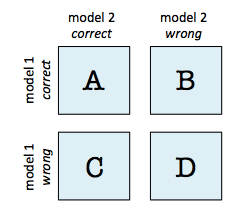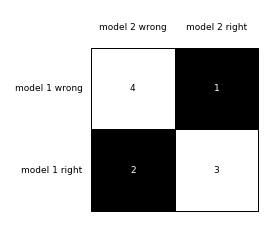# Contigency Tables for McNemar's Test and Cochran's Q Test

Function to compute a 2x2 contingency tables for McNemar's Test and Cochran's Q Test

from mlxtend.evaluate import mcnemar_tables

## Overview

### Contigency Tables

A 2x2 contigency table as being used in a McNemar's Test (mlxtend.evaluate.mcnemar) is a useful aid for comparing two different models. In contrast to a typical confusion matrix, this table compares two models to each other rather than showing the false positives, true positives, false negatives, and true negatives of a single model's predictions:For instance, given that 2 models have a accuracy of with a 99.7% and 99.6% a 2x2 contigency table can provide further insights for model selection.In both subfigure A and B, the predictive accuracies of the two models are as follows:

• model 1 accuracy: 9,960 / 10,000 = 99.6%
• model 2 accuracy: 9,970 / 10,000 = 99.7%

Now, in subfigure A, we can see that model 2 got 11 predictions right that model 1 got wrong. Vice versa, model 2 got 1 prediction right that model 2 got wrong. Thus, based on this 11:1 ratio, we may conclude that model 2 performs substantially better than model 1. However, in subfigure B, the ratio is 25:15, which is less conclusive about which model is the better one to choose.

## Example 1 - Single 2x2 Contigency Table

import numpy as np
from mlxtend.evaluate import mcnemar_tables

y_true = np.array([0, 0, 0, 0, 0, 1, 1, 1, 1, 1])

y_mod0 = np.array([0, 1, 0, 0, 0, 1, 1, 0, 0, 0])
y_mod1 = np.array([0, 0, 1, 1, 0, 1, 1, 0, 0, 0])

tb = mcnemar_tables(y_true,
y_mod0,
y_mod1)

tb

{'model_0 vs model_1': array([[ 4.,  1.],
[ 2.,  3.]])}


To visualize (and better interpret) the contigency table via matplotlib, we can use the checkerboard_plot function:

from mlxtend.plotting import checkerboard_plot
import matplotlib.pyplot as plt

brd = checkerboard_plot(tb['model_0 vs model_1'],
figsize=(3, 3),
fmt='%d',
col_labels=['model 2 wrong', 'model 2 right'],
row_labels=['model 1 wrong', 'model 1 right'])
plt.show()## Example 2 - Multiple 2x2 Contigency Tables

If more than two models are provided as input to the mcnemar_tables function, a 2x2 contingency table will be created for each pair of models:

import numpy as np
from mlxtend.evaluate import mcnemar_tables

y_true = np.array([0, 0, 0, 0, 0, 1, 1, 1, 1, 1])

y_mod0 = np.array([0, 1, 0, 0, 0, 1, 1, 0, 0, 0])
y_mod1 = np.array([0, 0, 1, 1, 0, 1, 1, 0, 0, 0])
y_mod2 = np.array([0, 0, 1, 1, 0, 1, 1, 0, 1, 0])

tb = mcnemar_tables(y_true,
y_mod0,
y_mod1,
y_mod2)

for key, value in tb.items():
print(key, '\n', value, '\n')

model_0 vs model_1
[[ 4.  1.]
[ 2.  3.]]

model_0 vs model_2
[[ 4.  2.]
[ 2.  2.]]

model_1 vs model_2
[[ 5.  1.]
[ 0.  4.]]


## API

mcnemar_tables(y_target, y_model_predictions)*

Compute multiple 2x2 contigency tables for McNemar's test or Cochran's Q test.

Parameters

• y_target : array-like, shape=[n_samples]

True class labels as 1D NumPy array.

• y_model_predictions : array-like, shape=[n_samples]

Predicted class labels for a model.

Returns

• tables : dict

Dictionary of NumPy arrays with shape=[2, 2]. Each dictionary key names the two models to be compared based on the order the models were passed as *y_model_predictions. The number of dictionary entries is equal to the number of pairwise combinations between the m models, i.e., "m choose 2."

For example the following target array (containing the true labels) and 3 models

• y_true = np.array([0, 0, 0, 0, 0, 1, 1, 1, 1, 1])
• y_mod0 = np.array([0, 1, 0, 0, 0, 1, 1, 0, 0, 0])
• y_mod0 = np.array([0, 0, 1, 1, 0, 1, 1, 0, 0, 0])
• y_mod0 = np.array([0, 1, 1, 1, 0, 1, 0, 0, 0, 0])

would result in the following dictionary:

{'model_0 vs model_1': array([[ 4., 1.], [ 2., 3.]]), 'model_0 vs model_2': array([[ 3., 0.], [ 3., 4.]]), 'model_1 vs model_2': array([[ 3., 0.], [ 2., 5.]])}

Each array is structured in the following way:

• tb[0, 0]: # of samples that both models predicted correctly
• tb[0, 1]: # of samples that model a got right and model b got wrong
• tb[1, 0]: # of samples that model b got right and model a got wrong
• tb[1, 1]: # of samples that both models predicted incorrectly

Examples

For usage examples, please see
[http://rasbt.github.io/mlxtend/user_guide/evaluate/mcnemar_tables/](http://rasbt.github.io/mlxtend/user_guide/evaluate/mcnemar_tables/)